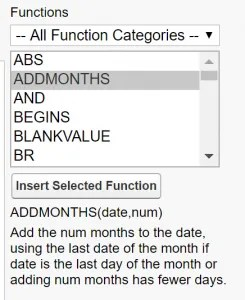# MCEILING

• ## Secrets of Spring ’18: Hidden Formulas

Spring ’18 is now live for most orgs, but there appears to be some changes included in the release which haven’t been documented :-O The great news for us Admins, the changes actually resolve a number of ‘simple’ problems we have when trying to calculate values in formulas.  So in this quick update we look at some of the new ‘hidden formulas’ included with Spring ’18 and some of the use cases where you might be able to apply them…

Update: 26/Feb  – Eagle-eyed Ekaterina has pointed out in the comments, there are even more additional formulas available!  Here is a link to her blog if you want to read more…

Update: 01/Mar – Salesforce have now scheduled an update to the Known Issue and scheduled an update via the Spring ’18 Patch 11.0.  To see what’s included, click here.The first of the hidden formulas included in Spring ’18 is the new AddMonths function.

Imagine you need a formula to calculate a date, which based off another date entered on a record.  For example, you could see this in action in Contract End Date (contract start date + 2mth duration = contract end date).

The formula to add 2 months, while only using point and click Admin features, is quite little long winded (if you want to accurately account for Leap Years):

```IF(
MOD( MONTH( date ) + 2, 12 ) = 2,
IF(
DAY( date ) > 28,
DATE( YEAR( date ) + FLOOR( ( MONTH( date ) + 2 ) / 12 ), 3, 1 ),
DATE( YEAR( date ) + FLOOR( ( MONTH( date ) + 2 ) / 12 ), 2, DAY( date ) )
),
IF(
OR(
MOD( MONTH( date ) + 2, 12 ) = 4,
MOD( MONTH( date ) + 2, 12 ) = 6,
MOD( MONTH( date ) + 2, 12 ) = 9,
MOD( MONTH( date ) + 2, 12 ) = 11
),
IF(
DAY( date ) > 30,
DATE( YEAR( date ) + FLOOR( ( MONTH(  date  ) + 2 ) / 12 ),
MOD( MONTH( date ) + 2, 12 ) + 1, 1 ),
DATE( YEAR( date ) + FLOOR( ( MONTH( date ) + 2 ) / 12 ),
MOD( MONTH( date ) + 2, 12), DAY( date ) )
),
IF(
MOD( MONTH( date ) + 2, 12 ) = 0,
DATE( YEAR( date ) + FLOOR( ( MONTH( date ) + 2 ) / 12 ) - 1, 12, DAY( date ) ),
DATE( YEAR( date ) + FLOOR( ( MONTH( date ) + 2 ) / 12 ),
MOD( MONTH( date ) + 2, 12), DAY( date ) )
)
)
)```

GULP!!

But, now you will be able to use the AddMonths formula…

So you want to add 2 months, to a field containing the start date of the contract, it is now as simple as:

`addMonths(date, 2)`

To see more details, until the official documentation is available, visit Ekaterina’s post here.

## Hidden Formulas: WEEKDAY

Also in Spring ’18, and a little more obscure, is the new WeekDay function.

It allows you to return a number (from 1-7) which indicates the day of the week, based on a date field within Salesforce.

Before, once again, you could retrieve this information – but it was again a bit cumbersome:

```CASE(
MOD( DATEVALUE(CreatedDate) - DATE(1900, 1, 7), 7),
0, "Sunday",
1, "Monday",
2, "Tuesday",
3, "Wednesday",
4, "Thursday",
5, "Friday",
6, "Saturday",
"Error"
)```

But now – simply use:

`WeekDay(date)`

And it will return for you, a number which represents the day of the week:

```1 = Sunday
2 = Monday
3 = Tuesday
4 = Wednesday
5 = Thursday
6 = Friday
7 = Saturday```

## Hidden Formulas: CURRENCYRATE

The next hidden formula in Spring ’18, is CURRENCYRATE.

This formula will return the conversion rate used for a record’s currency code.

So for example, if you have the default currency as USD.  But the record’s currency is GBP – you can now retrieve what the conversion rate is between those currencies.  And then display it as a formula field or use it in reports.

`CURRENCYRATE(IsoCode)`

As a possibly obvious tip, Currency Management will need to be activate in your org before you can use this…

The returned value will be a number showing the currency conversion rate, eg 0.717412 (but you will be able to control how many decimals are shown).

## Hidden Formulas: MFLOOR & MCEILING

These two final formula functions go hand in hand.  And now also available in Spring ’18!!

`Round(decimalNumber, 2)`

Where you could round a decimal number to the number of decimals you specified in the formula.  But you couldn’t force Salesforce to round up or down… Until now!

MCEILING will round a number up to the nearest positive whole number.  Whereas the MFLOOR will round down to the nearest positive whole number.

Both functions will return 0, if the input number is a negative.

So for example, if you have a number field which contains decimals but you want to round up or down, you can control this with these functions.

To round up, to nearest whole number:

`MCEILING(number)`

To round down, to nearest whole number:

`MFLOOR(number)`

# Found any others?

No doubt Salesforce will be updating all relevant documentation and the Help site soon.  But if you have found any others, add them to the comments below!